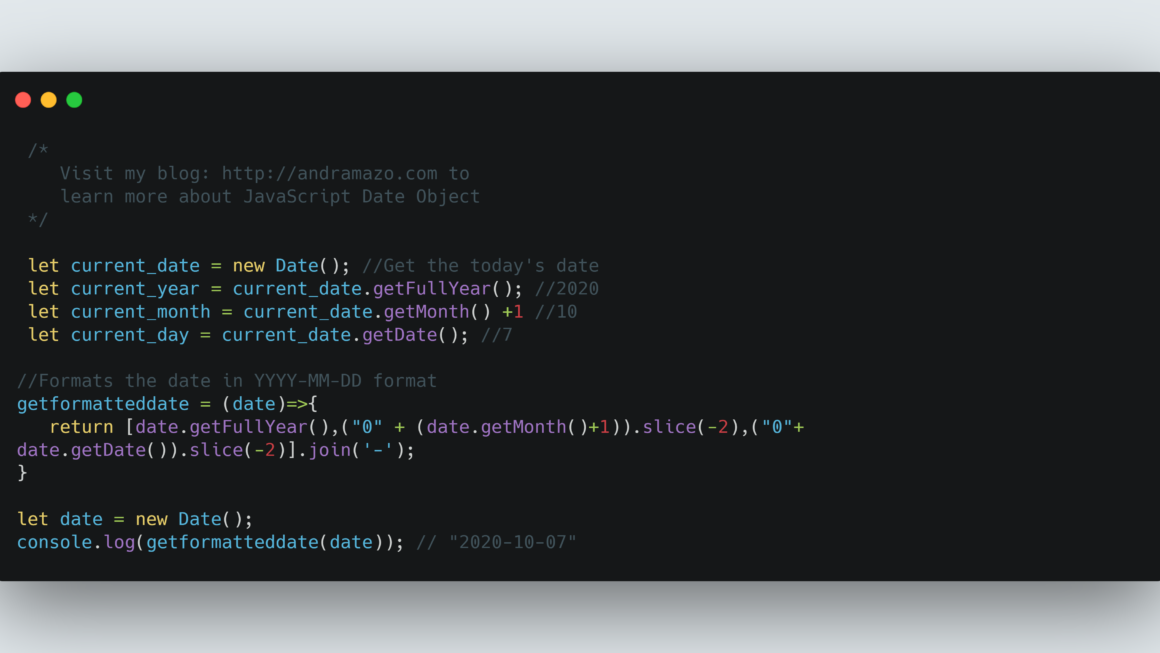# Playing with JavaScript DatesJavaScript Date objects are not the easiest to work with. This is why many developers install different modules such as moment.js, or Luxon.

Let’s say you would like to get the month, date and year from the provided Date object, you can fetch it as shown below. In JavaScript, getMonth() returns the month where January is 0 so to get the current month. We need to add 1 to it.

`````` let current_date = new Date(); //Get the today's date
let current_year = current_date.getFullYear(); //2020
let current_month = current_date.getMonth() +1 //10
let current_day = current_date.getDate(); //7``````

Now let us make a function which returns the Date object into YYYY-MM-DD format. Here, we will add ‘0’ before the month and date values so that for all the values which are single digits, they will be formatted perfectly into two digits. The example shows how to generate a date in YYYY-MM-DD format. Using this we can generate dates in the format of MM-YYYY, DD-MM-YYYY, DD-MM as well.

``````//Formats the date in YYYY-MM-DD format
getformatteddate = (date)=>{
return [date.getFullYear(),("0" + (date.getMonth()+1)).slice(-2),("0"+ date.getDate()).slice(-2)].join('-');
}

let date = new Date();
console.log(getformatteddate(date)); // "2020-10-07"``````

To find the day for the provided Date object, you can call getDay() where Sunday is 0, Monday is 1 and so on.

``````let date = new Date();
console.log(date.getDay()); //will provide current date's day.``````

Let us try to find the previous date from the provided date object. My current date is October 7th 2020.

`````` let current_date = new Date(); // Wed Oct 07 2020 20:24:26 GMT-0400 (Eastern Daylight
let vwap_date = new Date(new Date().setDate(current_date.getDate() - 1)); //Will return Tue Oct 06 2020 20:24:26 GMT-0400 (Eastern Daylight Time)``````